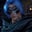Related Tags

julia

# How to get the determinant of a matrix in JuliaGutha Vamsi Krishna

### Overview

In this shot, we'll learn how to get the determinant of a matrix using Julia. The determinant of a matrix is the scalar value computed for a given square matrix.

We can get the determinant of a matrix in Julia using the function det() provided by the LinearAlgebra package.

### Syntax

det(matrix)

### Parameter and return value

The det() function takes a matrix as a parameter and returns its scalar value.

Let us take a look at an example of this.

### Example

using LinearAlgebra

#given matrix
A = [1 -1; 0 2]

#get determinant of matrix
display(det(A))

### Explanation

In the code snippet above:

• In line 1 we import the LinerAlgebra package to use the det() function.
• In line 4 we declare and initialize a matrix A.
• In line 7 we get the determinant of the matrix A using the det() function and display the resultant scalar value.

RELATED TAGS

julia

CONTRIBUTORGutha Vamsi Krishna
RELATED COURSES

View all Courses

Keep Exploring

Learn in-demand tech skills in half the time